## CodeNewbie Community is a community of 4,499 amazing newbies# Variable arguments in Java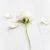Abhann
Learning || Opinions can change over the course of exposure to new info...

In this article, you will learn about Varargs in Java, when to use and when not to use them and how the concept is useful for a programmer. A shorter name for variable arguments in Java is varargs.
An argument of a method can accept a random number of values, this argument that can accept an arbitrary number of values is varargs.

The syntax for implementing variable arguments is as follows;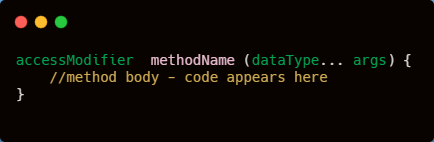As you can see above, in order to define varargs, “…” the three dots in the formal parameter of a method is key. A method that takes a variable number of arguments is a variable-arity method, or simply a varargs method.
Before moving on, let’s look at an example without using varargs method, and also please see my article on Variables for a comprehensive insight on methods.

Let’s see how we can use this core Java feature.

### Variable arguments in Java

Let’s suppose you are creating a method in Java; It is good coding practise to specify the arguments it accepts along with its data type as seen below.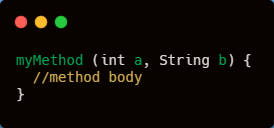But suppose you are not sure of how many arguments your method is going to accept, or you need to accept over one variable of the same type, you will then need to specify the variables one after the other as;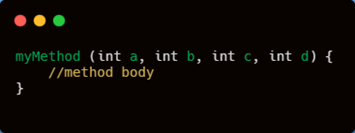This is where Varargs comes in, as you can see the second example is lengthy and considered a poor programming practice, varargs will help optimize the syntax usage such that we can specify even more than the required variable if need be.
Let’s take an example using a method that finds the sum of numbers, without the help of varargs.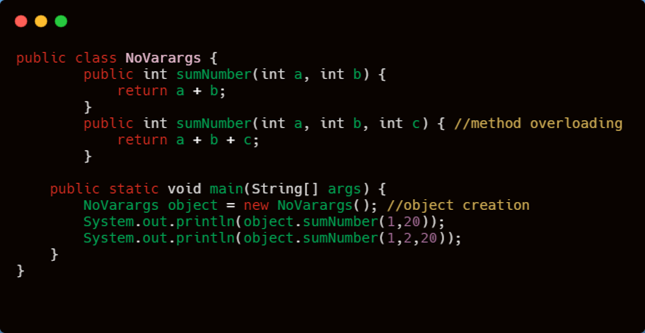When you run this program, the output will be;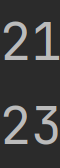The first method accepts two arguments and as we can see, I had to overload the sumNumber() method to make it accommodate three arguments, after sometime, say now, my new requirement is the sum of ten values, I cannot re-use the method above anymore, because it clearly applies for only three values and can take only three values.
Another thing we can deduct from above is, this system increases the length of code and also reduces readability.

If there’s ever a change in the number of arguments(values), then we must always go for a new method and so on. Declaring multiple sumNumber() automatically reduces the readability of the code.

So some people analyzed this problem, and in the version 1.5, they made it possible to not need to take that many “sumNumber()”, only one method is needed and we can call it by passing many int values, as many as you like.

We call such type of methods Variable-Argument, and so thanks to introducing varargs, I can handle this neatly, such that our code is simple and easy to understand.

Our Output would result;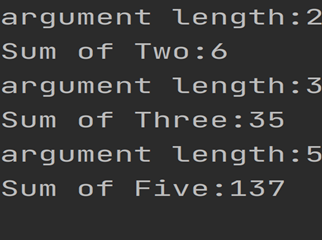Above, the sumNumber() method returns the sum of int parameters passed to it, and as we can see, it can accept any amount of arguments.

Let’s take two more examples;

Observe again;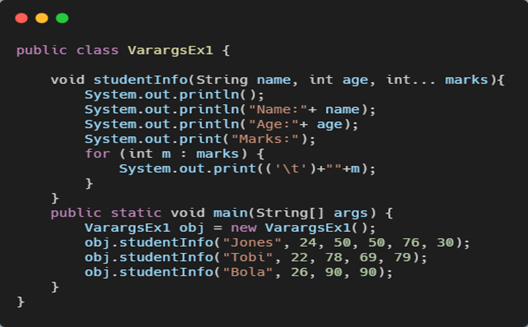Here, we pass three different data types into the parameter of the studentInfo() method, the “…” syntax tells the Java compiler that the method can be called with zero or more arguments. As a result, “marks” variable is implicitly declared as an array of type “int[]”. Thus inside the method, “marks” variable is accessed using the array syntax and at the output we get;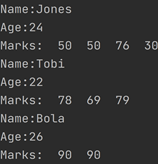In case of no arguments, the length of marks is 0.

As we move on, we see how varargs can be really useful in some situations. Once you use variable arguments as a parameter method while calling a method, you can pass as many number of arguments or even call it passing no argument.

However, if you are certain about the number of arguments passed to a method, use method overloading instead. Varargs are basically like arrays, so we need to work with them just like we’d work with a normal array.

Please read my article on ARRAYS, for a recap on how arrays work.

Hence, the twist is, internally, the vararg parameter will be converted into 1 - D Arrays, however wherever a vararg parameter is present, it can’t be replaced with the 1–D Array, and within the var-arg method, we can differentiate values by using index.

There are some loopholes and ambiguities to be careful of when working with variable arguments, and they are as follows.

Case 1

We can mix var-arg parameters with normal parameters.

As seen above, while we defined the method, we kept varargs at last, the variable argument must be the last argument passed to the method, if you cannot do it this way, the compiler cannot figure out the number of arguments passed to the method, and you will get a compile error.

What happens is the Java compiler assigns the first argument to “X”, and the remaining “int” arguments are assigned to “Y”.

Case 2

Inside a varargs method, we can take one vararg parameter only.

Ex;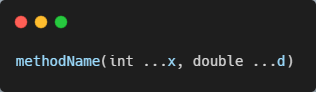That makes this method declaration incorrect, and a compile error follows.

Case 3

Above, the compiler gets confused if you try to invoke the random() even though it has been overloaded and now accepts a different number of arguments.

The compiler doesn’t know which method to call, because the two methods have the same name “random()”, and since there’s two possibilities, it causes ambiguity. Because of this, sometimes you may need to use different method names instead of overloading the varargs method.

Var-arg method will get the least priority, if no other method matches with a request then only var-arg method will get the chance, It is exactly the same as the default case inside “switch” statements.

In Java, if there’s any case where the IDE has to choose between an old concept and a new one, the old concept is always going to have priority, to provide compatibility with the old versions, they arranged it in that manner.

Equivalence between var-arg parameter and 1 – D Array.

The declaration;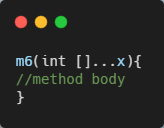is valid, and we can call this method by passing a group of 1 – D int Array, and “x” becomes a 2 – D int Array.

Let’s study the example below;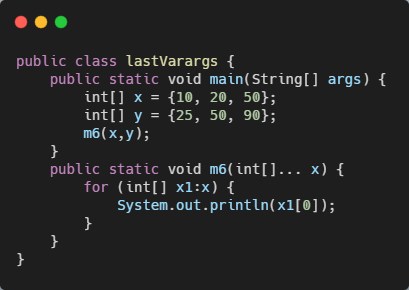Here, I took two 1 – D arrays of x and y, and I called m6() by passing the two arrays in as arguments in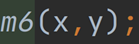, and yes the m6() is valid, as we can see, we can call it by passing a group of 1 – D Arrays, and we passed two.

X parameter in the m6() contains 1 – D Arrays and each 1 – D arrays contains three int values, as we can see.

In conclusion, using varargs can lead to “Heap Pollution”, but we won’t expand on that, mostly because varargs usage is safe if we use them to transfer a variable number of arguments from the caller to the method and nothing more!.

Varargs can make a lot of boilerplate syntax go away in Java, and thanks to their implicit auto-boxing to and from Arrays, they play a role in future–proofing our code, making it efficient.

## Discussion (2)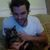Michael Tharrington (he/him)

This an awesome series. Thanks so much for sharing these!Abhann

thank you @Michael Tharrington, I am glad you find it interesting